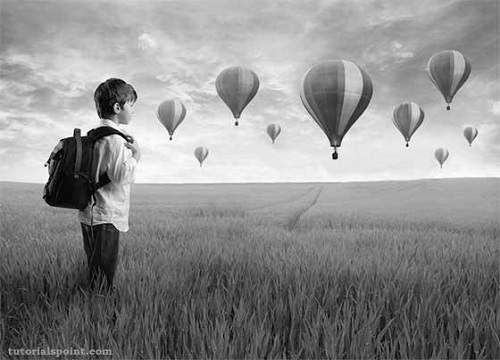# Java DIP - Understand Convolution

Convolution is a mathematical operation on two functions f and g. The function f and g in this case are images, since an image is also a two dimensional function.

## Performing Convolution

In order to perform convolution on an image, following steps are taken −

• Flip the mask (horizontally and vertically) only once.
• Slide the mask onto the image.
• Multiply the corresponding elements and then add them.
• Repeat this procedure until all values of the image has been calculated.

We use OpenCV function filter2D to apply convolution to images. It can be found under Imgproc package. Its syntax is given below −

```filter2D(src, dst, depth , kernel, anchor, delta, BORDER_DEFAULT );
```

The function arguments are described below −

Sr.No. Argument & Description
1

src

It is source image.

2

dst

It is destination image.

3

depth

It is the depth of dst. A negative value (such as -1) indicates that the depth is the same as the source.

4

kernel

It is the kernel to be scanned through the image.

5

anchor

It is the position of the anchor relative to its kernel. The location Point (-1, -1) indicates the center by default.

6

delta

It is a value to be added to each pixel during the convolution. By default it is 0.

7

BORDER_DEFAULT

We let this value by default.

### Example

The following example demonstrates the use of Imgproc class to perform convolution on an image of Grayscale.

```import org.opencv.core.Core;
import org.opencv.core.CvType;
import org.opencv.core.Mat;

import org.opencv.highgui.Highgui;
import org.opencv.imgproc.Imgproc;

public class convolution {
public static void main( String[] args ) {

try {
int kernelSize = 3;

Mat destination = new Mat(source.rows(),source.cols(),source.type());

Mat kernel = new Mat(kernelSize,kernelSize, CvType.CV_32F) {
{
put(0,0,0);
put(0,1,0);
put(0,2,0);

put(1,0,0);
put(1,1,1);
put(1,2,0);

put(2,0,0);
put(2,1,0);
put(2,2,0);
}
};

Imgproc.filter2D(source, destination, -1, kernel);
Highgui.imwrite("original.jpg", destination);

} catch (Exception e) {
System.out.println("Error:" + e.getMessage());
}
}
}
```

### Output

In this example we convolve our image with the following filter(kernel). This filter results in producing original image as it is −

 0 0 0 0 1 0 0 0 0

#### Original Image#### Convolved Image